# 2.1 Fill in the blanks (a) The volume of a cube of side 1 cm is equal to.....m3 (b) The surface area of a solid cylinder of radius 2.0 cm and height 10.0 cm is equal to ... (mm)2 (c) A vehicle moving with a speed of 18 km h.1 covers....m in 1 s (d) The relative density of lead is 11.3. Its density is ....g cm.3 or ....kg m.3.10 years ago

(a) 1 cm =

Volume of the cube = 1 cm3

But, 1 cm3 = 1 cm × 1 cm × 1 cm =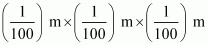∴1 cm3 = 10–6 m3

Hence, the volume of a cube of side 1 cm is equal to 10–6 m3.

(b) The total surface area of a cylinder of radius r and height h is

S = 2πr (r + h).

Given that,

r = 2 cm = 2 × 1 cm = 2 × 10 mm = 20 mm

h = 10 cm = 10 × 10 mm = 100 mm

= 15072 = 1.5 × 104 mm2

(c) Using the conversion,

1 km/h =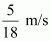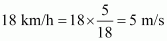Therefore, distance can be obtained using the relation:

Distance = Speed × Time = 5 × 1 = 5 m

Hence, the vehicle covers 5 m in 1 s.

(d) Relative density of a substance is given by the relation,

Relative density =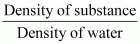Density of water = 1 g/cm3

Again, 1g =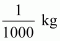1 cm3 = 10–6 m3

1 g/cm3 =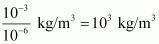∴ 11.3 g/cm3 = 11.3 × 103 kg/m3